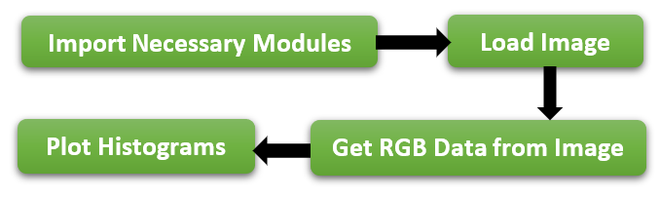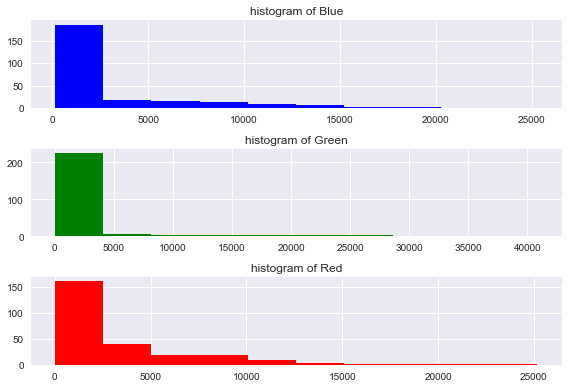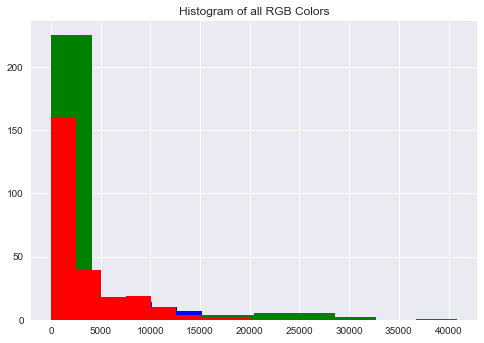# Visualizing Colors in Images Using Histogram in Python

• Last Updated : 18 Jan, 2022

In this article, we will discuss how to visualize colors in an image using histogram in Python.

An image consists of various colors and we know that any color is a combination of Red, Green, Blue. So Image consists of Red, Green, Blue colors. So using Histogram we can visualize how much proportion we are having RGB colors in a picture.

Histogram actually provides how frequently various colors occur in an image but not the location of color in an image. To visualize colors in the image we need to follow the below steps-## Stepwise Implementation

### Step 1: Import Necessary Modules

To this Concept mainly we need 2 modules.

• cv2It is used to load the image and get the RGB data from the image.
• matplotlibUsed to plot the histograms.

To load an image we need to use imread() method which is in the cv2 module. It accepts the image name as a parameter.

Syntax :

### Step 3: Get RGB Data from Image

To get the RGB colors from the image, the cv2 module provides calchist method that accepts the image object, channel to get a specific color, mask, histogram size, and range.

Syntax:

#### Parameters:

• imageObject- Variable name in which image is loaded.
• channelValue- It accepts 0,1,2 values. It helps us to get the required color.
• 0 indicates blue, 1 indicates red, 2 indicates green.

### Step 4: Plot histograms

matplotlib provides the hist method which is used to draw the histogram on specified data.

Syntax:

matplotlib.hist(data,color=”value”)

Example:

As per the above steps, First imported the required modules, and next we loaded an image using imread() method and using calcHist() method to get the RGB colors from Image and then plot the Histograms using the RGB data.

## Python3

 `# import necessary packages` `import` `cv2` `import` `matplotlib.pyplot as plt`   `# load image` `imageObj ``=` `cv2.imread(``'SampleGFG.jpg'``)` `# to avoid grid lines` `plt.axis(``"off"``)` `plt.title(``"Original Image"``)` `plt.imshow(cv2.cvtColor(imageObj, cv2.COLOR_BGR2RGB))` `plt.show()`   `# Get RGB data from image` `blue_color ``=` `cv2.calcHist([imageObj], [``0``], ``None``, [``256``], [``0``, ``256``])` `red_color ``=` `cv2.calcHist([imageObj], [``1``], ``None``, [``256``], [``0``, ``256``])` `green_color ``=` `cv2.calcHist([imageObj], [``2``], ``None``, [``256``], [``0``, ``256``])`   `# Separate Histograms for each color` `plt.subplot(``3``, ``1``, ``1``)` `plt.title(``"histogram of Blue"``)` `plt.hist(blue_color, color``=``"blue"``)`   `plt.subplot(``3``, ``1``, ``2``)` `plt.title(``"histogram of Green"``)` `plt.hist(green_color, color``=``"green"``)`   `plt.subplot(``3``, ``1``, ``3``)` `plt.title(``"histogram of Red"``)` `plt.hist(red_color, color``=``"red"``)`   `# for clear view` `plt.tight_layout()` `plt.show()`   `# combined histogram` `plt.title(``"Histogram of all RGB Colors"``)` `plt.hist(blue_color, color``=``"blue"``)` `plt.hist(green_color, color``=``"green"``)` `plt.hist(red_color, color``=``"red"``)` `plt.show()`

OutputMy Personal Notes arrow_drop_up
Recommended Articles
Page :